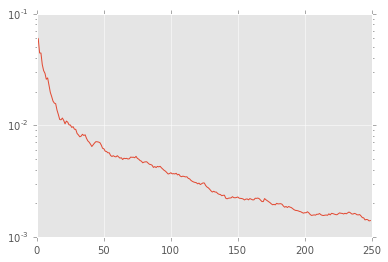# Noisy Coin Example¶

## Preamble¶

We turn on the division feature first of all, since this is just a plain good idea when working in Python 2.x, as it is a more sensible default for scientific computing and is the default in Python 3.x anyway.

In :
from __future__ import division, print_function


To get access to NumPy and matplotlib, IPython's %pylab magic command is quite useful. With the inline argument, all plots will be made a part of the notebook.

In :
%matplotlib inline
import numpy as np
import matplotlib.pyplot as plt
try: plt.style.use('ggplot')
except: pass

/home/cgranade/anaconda/envs/qinfer-binder/lib/python3.5/site-packages/matplotlib/__init__.py:872: UserWarning: axes.color_cycle is deprecated and replaced with axes.prop_cycle; please use the latter.
warnings.warn(self.msg_depr % (key, alt_key))


Next, we need to import from Qinfer.

In :
# We need distributions to model priors.
from qinfer import distributions
# The noisy coin model has already been implmented, so let's import it here.
from qinfer.test_models import NoisyCoinModel
# Next, we need to import the sequential Monte Carlo updater class.
from qinfer.smc import SMCUpdater
# We'll be demonstrating approximate likelihood evaluation (ALE) as well.
from qinfer import ale

/home/cgranade/anaconda/envs/qinfer-binder/lib/python3.5/site-packages/qinfer/metrics.py:51: UserWarning: Could not import scikit-learn. Some features may not work.
warnings.warn("Could not import scikit-learn. Some features may not work.")
/home/cgranade/anaconda/envs/qinfer-binder/lib/python3.5/site-packages/IPython/parallel.py:13: ShimWarning: The IPython.parallel package has been deprecated. You should import from ipyparallel instead.
"You should import from ipyparallel instead.", ShimWarning)
/home/cgranade/anaconda/envs/qinfer-binder/lib/python3.5/site-packages/qinfer/parallel.py:53: UserWarning: Could not import IPython parallel. Parallelization support will be disabled.
"Could not import IPython parallel. "

In :
import time
from scipy.special import betaln, gammaln


## Analytic Solution¶

In this case, we can compare to the analytic solution derived by Christopher Ferrie [cite].

In :
def exactBME(k, K, a, b, gamma=1):
idx_k = np.arange(k+1)
idx_K = np.arange(K-k+1)[np.newaxis].transpose()

numerator   = (
gammaln(k+1) - gammaln(idx_k+1) - gammaln(k-idx_k+1) + gammaln(K-k+1) -
gammaln(idx_K+1) - gammaln(K-k-idx_K+1) + (idx_k+idx_K)*np.log(a-b) +
(k-idx_k)*np.log(b) + (K-k-idx_K)*np.log(1-a) +
betaln(idx_k+gamma+1,idx_K+gamma)
)
denominator = (
gammaln(k+1) - gammaln(idx_k+1) - gammaln(k-idx_k+1) + gammaln(K-k+1) -
gammaln(idx_K+1) - gammaln(K-k-idx_K+1) + (idx_k+idx_K)*np.log(a-b) +
(k-idx_k)*np.log(b) + (K-k-idx_K)*np.log(1-a) +
betaln(idx_k+gamma,idx_K+gamma)
)
bme = np.sum(np.exp(numerator))/np.sum(np.exp(denominator))

var = np.sum(np.exp(
numerator - betaln(idx_k+gamma+1,idx_K+gamma) +
betaln(idx_k + gamma + 2, idx_K + gamma)
)) / np.sum(np.exp(denominator)) - bme ** 2

return bme, var


## Sequential Monte Carlo¶

It's helpful to define a few constants, as we'll need to refer to them over and over below.

In :
N_PARTICLES = 5000
N_EXP = 250
N_TRIALS = 100


Let's make a model to play with, using the prior $p \sim \mathrm{Uni}(0, 1)$.

In :
prior = distributions.UniformDistribution([0, 1])
model = NoisyCoinModel()


We need to allocate an array to hold performance data. A record array is a rather convienent structure for doing so. First, let's define the fields in this array,

In :
performance_dtype = [
('outcome', 'i1'),
('est_mean', 'f8'), ('est_cov_mat', 'f8'),
('true_err', 'f8'), ('resample_count', 'i8'),
('elapsed_time', 'f8'),
('like_count', 'i8'), ('sim_count', 'i8'),
('bme', 'f8'),
('var', 'f8'),
('bme_err', 'f8')
]


... and then the array itself.

In :
performance = np.empty((N_TRIALS, N_EXP), dtype=performance_dtype)

In :
true_params = np.empty((N_TRIALS, model.n_modelparams))


Now, we run the experiments!

In :
ALPHA = 0.1
BETA = 0.8
expparams = np.array([(ALPHA, BETA)], dtype=model.expparams_dtype)

In :
for idx_trial in range(N_TRIALS):

# First, make new updaters using the constructors
# defined above.
updater = SMCUpdater(model, N_PARTICLES, prior)

# Sample true set of modelparams.
truemp = prior.sample()
true_params[idx_trial, :] = truemp

# Now loop over experiments, updating each of the
# updaters with the same data, so that we can compare
# their estimation performance.
for idx_exp in range(N_EXP):

# Make a short hand for indexing the current simulation
# and experiment.
idxs = np.s_[idx_trial, idx_exp]

# Start by simulating and recording the data.
outcome = model.simulate_experiment(truemp, expparams)
performance['outcome'][idxs] = outcome

# Reset the like_count and sim_count
# properties so that we can count how many were used
# by this update. Note that this is a hack;
# an appropriate method should be added to
# Simulatable.
model._sim_count = 0
model._call_count = 0

# Time the actual update.
tic = toc = None
tic = time.time()
updater.update(outcome, expparams)
performance[idxs]['elapsed_time'] = time.time() - tic

# Record the performance of this updater.
est_mean = updater.est_mean()
performance[idxs]['est_mean'] = est_mean
performance[idxs]['true_err'] = np.abs(est_mean - truemp) ** 2
performance[idxs]['est_cov_mat'] = updater.est_covariance_mtx()
performance[idxs]['resample_count'] = updater.resample_count
performance[idxs]['like_count'] = model.call_count
performance[idxs]['sim_count'] = model.sim_count

# Finally, record the ideal stats.
performance[idxs]['bme'], performance[idxs]['var'] = exactBME(
idx_exp + 1 - np.sum(performance[idxs]['outcome']), idx_exp + 1,
ALPHA, BETA
)
performance[idxs]['bme_err'] = np.abs(performance[idxs]['bme'] - truemp) ** 2

/home/cgranade/anaconda/envs/qinfer-binder/lib/python3.5/site-packages/ipykernel/__main__.py:8: RuntimeWarning: invalid value encountered in log
/home/cgranade/anaconda/envs/qinfer-binder/lib/python3.5/site-packages/ipykernel/__main__.py:14: RuntimeWarning: invalid value encountered in log

In :
plt.semilogy(np.mean(performance['true_err'], axis=0))

Out:
[<matplotlib.lines.Line2D at 0x7ff45cb02588>]In [ ]: